Perimeter of Compound Shapes
starstarstarstarstarstarstarstarstarstar
4.5 (1 rating)
by Felix Gabathuler
| 13 Questions# Let's warm up with a coupla quick ones. If you need a refresher on how to find circumference, watch the following video. If not, skip it and start answerin' those questions!1
1
Bicycles in the late 1800s looked very different than they do today, as you can see by the picture above. Using our knowledge of circumference, calculate how many rotations the front tire makes after travelling 600 feet? (Remember, 12 inches = 1 foot)(Round your answer to the nearest whole number) (Do not include units in your answer)
2
1
Using the picture above, how many rotations does the smaller back tire make if you travelled 600 feet? (Remember, 12 inches = 1 foot)(Round your answer to the nearest whole number) (Do not include units in your answer)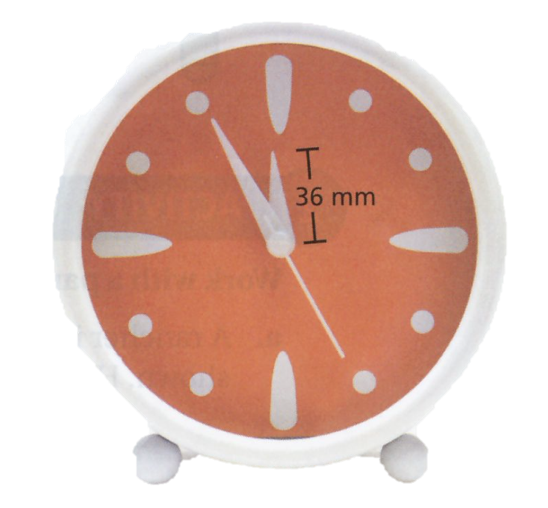3
1
The length of the minute had above is 150% of the length of the hour hand. Think about that for a second.... ok. Let's move on. What distance will the tip of the minute hand move in 45 minutes? Round your answer to the nearest whole number.
4
1
In 1 hour, how much farther does the tip of the minute hand move than the tip of the hour hand? Round your answer to the nearest whole number and don't include units.# - Your cost for the tile is \$4 per linear foot

5
1
How many brown tiles do you need for the border?
6
1
Is there an easier way to figuring out how many tiles you need besides counting each individual tile? Explain your answer.# To find the perimeter of a composite shape, you find the distance around the figure.7
1
What is the perimeter of the compound shape above. Do not include the units.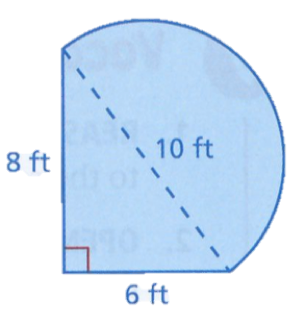8
1
Find the perimeter of the shape above. Round your final answer to the nearest tenth of a foot.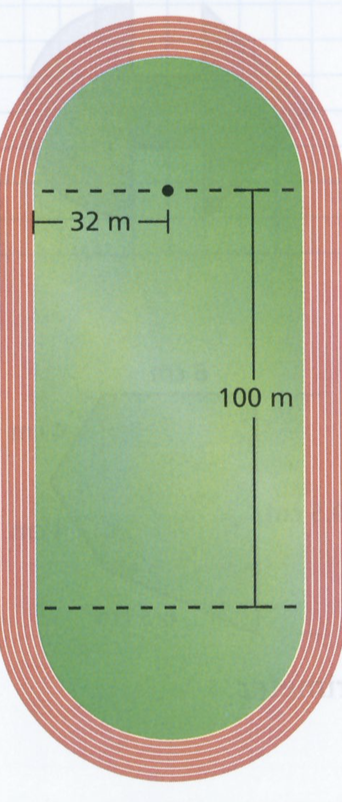9
1
The running track above is made up of a rectangle and two semicricles. Find the perimeter. Round your answer to the nearest hundredth of a meter.10
1
Find the perimeter of the figure above. Round your answers to the nearest tenth of a foot.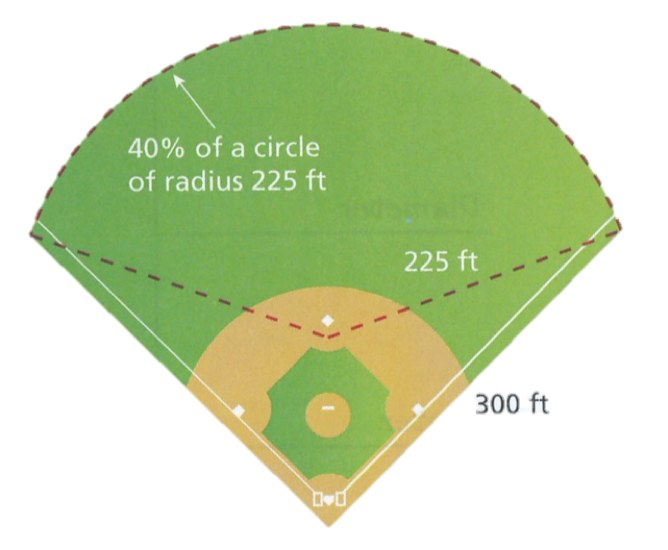11
1
You run around the perimeter of the baseball field above at a rate of 9 feet per second. How long does it take you to run around the baseball field? Give your answer in minutes and round to the nearest minute.
12
1
Design a pool that has a composite shape consisting of part of a circle, triangle, and quadrilateral. In the space below, draw your design. Then find the perimeter of the pool. Include relevant measurements. If you want, you can draw the design on a sheet of paper or Geogebra and upload a picture.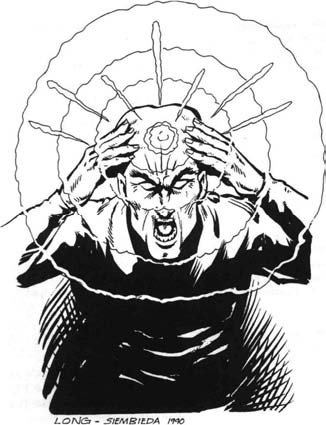13
1
How can you add a figure to a composite figure without increasing its perimeter? Draw a diagram to support your answer.